基于 HTML5 WebGL 的发动机 3D 可视化系统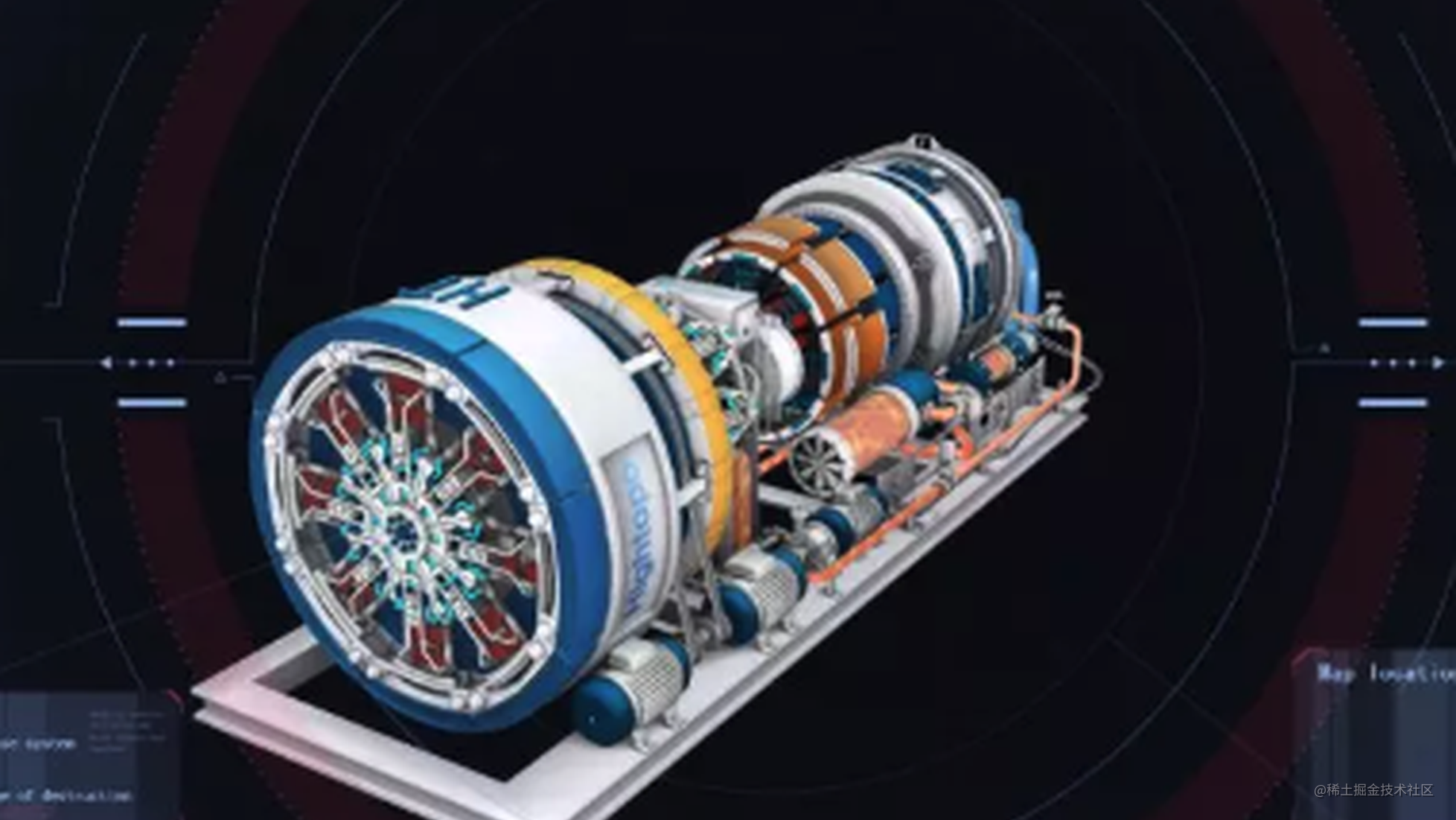http://www.hightopo.com/demo/engine/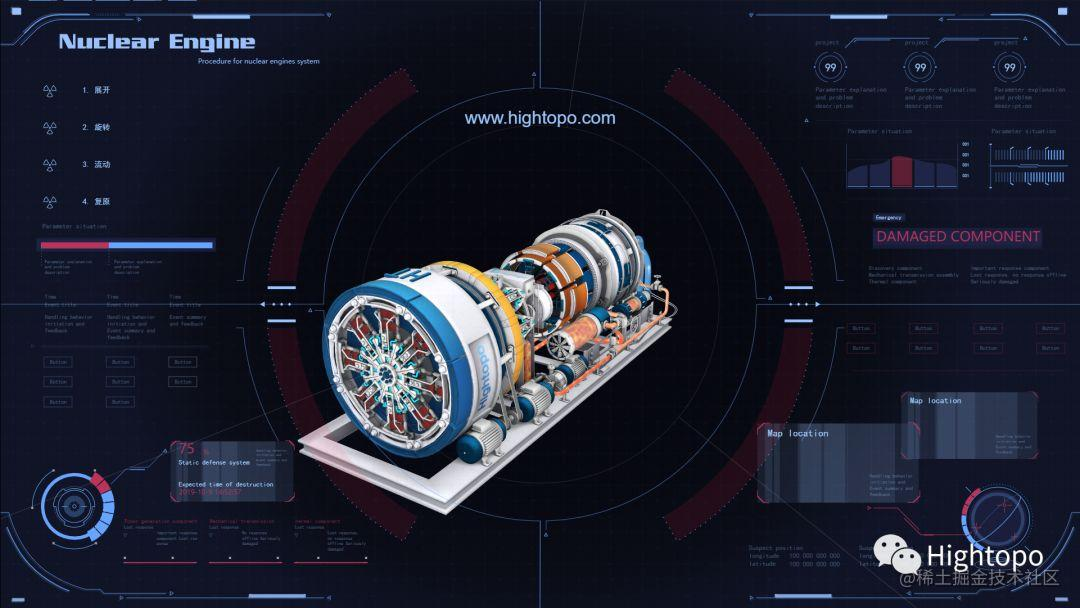2D 面板部分则是通过 HT 的矢量绘制，我们需要创建 ht.graph.GraphView 和 ht.graph3d.Graph3dView 来呈现 2D 和 3D 的内容。相关代码如下：

var g2d = new ht.graph.GraphView();var g3d = new ht.graph3d.Graph3dView();// 将 3D 组件加入到 body 下g3d.addToDOM();// 将 2D 组件加入到 3D 组件的根 div 下g2d.addToDOM(g3d.getView());复制代码

设备拆解动画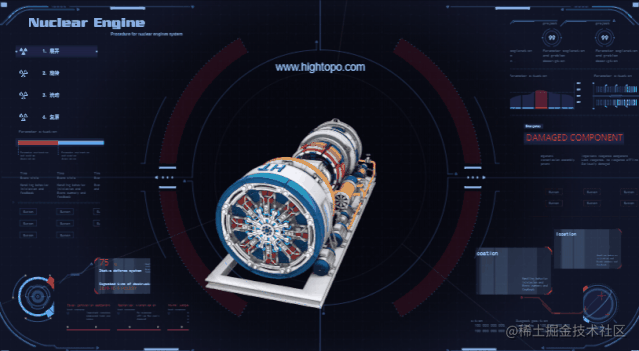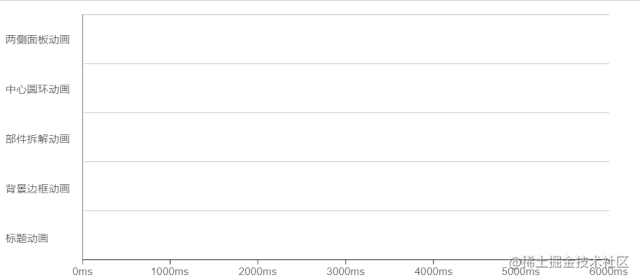// 圆环动画animCenter(data) {    ht.Default.startAnim({        duration: 4500,        // 设置缓动函数        easing: Easing.backBoth,        // 延迟一秒执行动画        delay: 1000,        action: function (v, t) {            // 修改图元缩放值            data.setScale(1 + v * 0.9, 1 + v * 0.9);            // 修改图元旋转角度            data.setRotation(Math.PI * v);        },    });}// 缓动函数Easing.easeOutStrong = function (t) {    var BACK_CONST = 1.70158;    if ((t *= 2) < 1) {        return .5 * (t * t * (((BACK_CONST *= (1.525)) + 1) * t - BACK_CONST));    }    return .5 * ((t -= 2) * t * (((BACK_CONST *= (1.525)) + 1) * t + BACK_CONST) + 2);};复制代码

// targetP3 为拆解后的坐标，p3为当前坐标var p3 = node.p3();var offset = [targetP3 - p3, targetP3 - p3, targetP3 - p3];// targetR3 为拆解后的旋转角度，r3为当前旋转角度let r3 = node.r3();let offset = [targetR3 - r3, targetR3 - r3, targetR3 - r3];// 拆解动画ht.Default.startAnim({    duration: 2000,    // 设置缓动函数    easing: Easing.easeOutStrong,    delay: 1000,    action: function (v, t) {        // 修改节点坐标        node.p3(p3 + offset * v, p3 + offset * v, p3 + offset * v);        // 修改节点旋转角度        node.r3(r3 + offset * v, r3 + offset * v, r3 + offset * v);    },});// 缓动函数Easing.easeOutStrong = function (t) {    return 1 - (--t) * t * t * t;};复制代码

// 设置图元裁剪方向为从右到左node.s('clip.direction', 'left');// 裁剪动画ht.Default.startAnim({    duration: 1800,    easing: Easing.easeOutStrong,    action: function (v, t) {        // 修改图元裁剪比例        node.s('clip.percentage', 1 - v);    }});// 缓动函数Easing.easeOutStrong = function (t) {    return 1 - (--t) * t * t * t;};复制代码

设备内部运作动画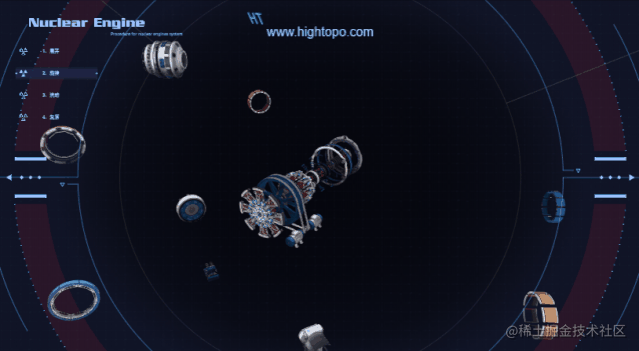g3d.moveCamera([-466, 93, -280], [40, -40, -40], {    duration: 2500,    easing: function (t) {        return 1 - (--t) * t * t * t;    },});复制代码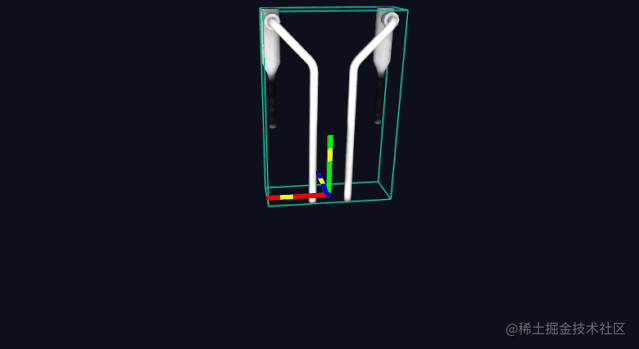ht.Default.startAnim({    duration: 800,    action: function (v, t) {        // 修改节点 Y 轴锚点        node.setAnchor3d(0.5,v,0.5);    },};复制代码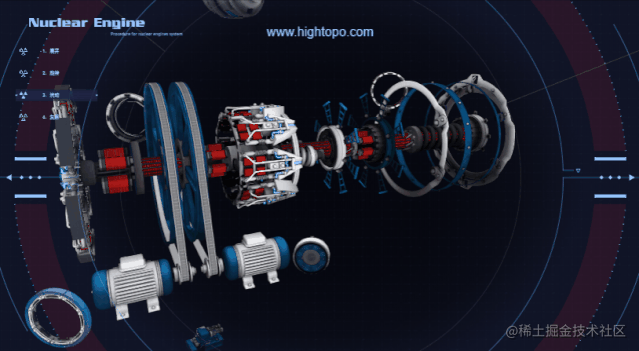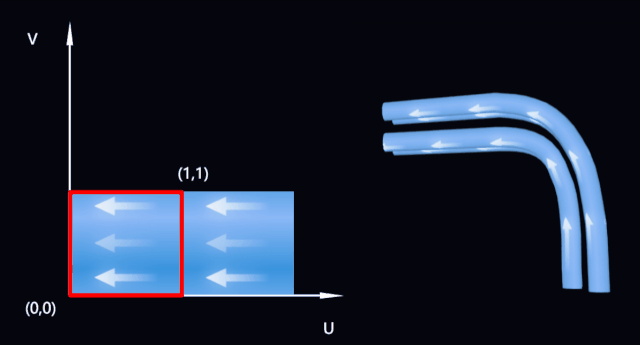代码如下：

ht.Default.startAnim({    duration: 2000,    action: function (v, t) {        // 修改贴图uv值        node.s('shape3d.uv.offset', [v, 0]);    },};复制代码

设备复原动画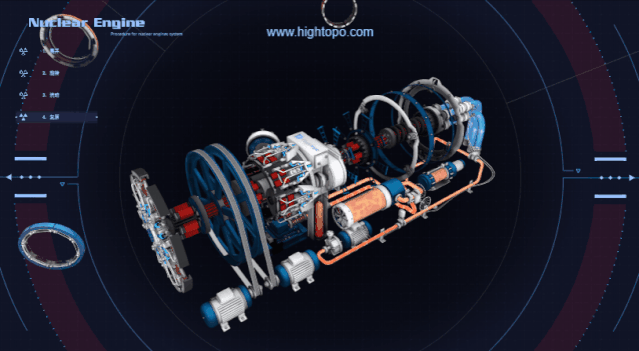鼠标移入效果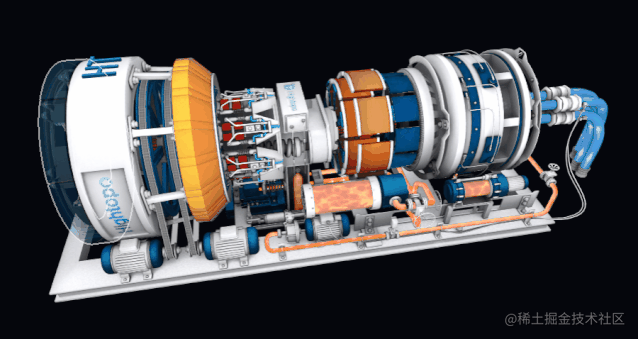// 设置高亮颜色ht.Style['highlight.color'] = 'rgba(255,255,255,0.6)';// 设置当前高亮的模式g3d.setHighlightMode('mouseover');// 节点启用高亮效果data.s('highlight.visible', true);// 节点设置为可交互data.s('interactive', true);// 节点开启交互后，不阻止场景上默认的交互行为data.s('preventDefaultWhenInteractive', false);// 监听交互事件g3d.mi(function (e) {    // 鼠标移入事件    if (e.kind === 'onEnter') {        // 节点开启透明        data.s('shape3d.transparent', true);        // 设置节点透明度        data.s('shape3d.opacity', 0.25);    }    // 鼠标移出事件    if (e.kind === 'onLeave') {        data.s('shape3d.transparent', false);    }});复制代码

场景视角限制

// 设置最大角度ht.Default.graph3dViewMaxPhi = Math.PI * 1 / 2;// 设置最小角度ht.Default.graph3dViewMinPhi = Math.PI * 1 / 4;复制代码